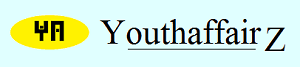﻿ Youthaffairz | Teaser: Find their agesOctober 2015

# Solution

Let's represent the ages of the two boys as x and y. So, the boys are standing in the following ascending order according to their ages: 6, 9, x, y, 13.

The age of the boy standing in the middle is x.

The average age of the five boys is (6 + 9 + x + y + 13)/5 = (28 + x + y)/5.

The age of the boy standing in the middle is equal to the average age. Therefore, x = (28 + x + y)/5.

Therefore, 5x = 28 + x + y.

So, 4x = 28 + y.

The boys are standing in an ascending order of their ages and no two boys are of the same age. Therefore, the ages of the two boys have to be greater than 9 and less than 13. But both the boys celebrated their birthdays today and, therefore, their ages have to be exact years (positive integers) and not fractions. Thus, they cannot be, for instance, 11.5 years or something like that.

The positive integers greater than 9 and less than 13 are 10, 11 and 12.

Now x cannot be 12 because if x is equal to twelve then y is either 10 or 11 and we don't get an ascending order. Thus, x is either 10 or 11.

Let's consider x = 11. We have 4x = 28 + y. Therefore, 44 = 28 + y. So, y = 16. This doesn't work out because y has to be one of the two remaining numbers 10 or 12.

The obvious choice therefore is x = 10. So, now we have 40 = 28 + y. Thus y = 12. That's fine because all the conditions are satisfied. Therefore, x = 10 and y = 12. So, the boys are standing in the following ascending order according to their ages: 6, 9, 10, 12, 13. Did I get it right or did I make a mistake as usual?

• Union Public Service Commission - www.upsc.gov.in
• IIT-Kharagpur - www.iitkgp.ac.in
• Indian Statistical Institute - www.isical.ac.in
• Indian Institute of Technology Madras - www.iitm.ac.in
• Indian Institute of Management, Ahmedabad - www.iimahd.ernet.in
• Indian Institute of Mass Commission - www.iimc.nic.in
• IIT Bombay - www.iitb.ac.in